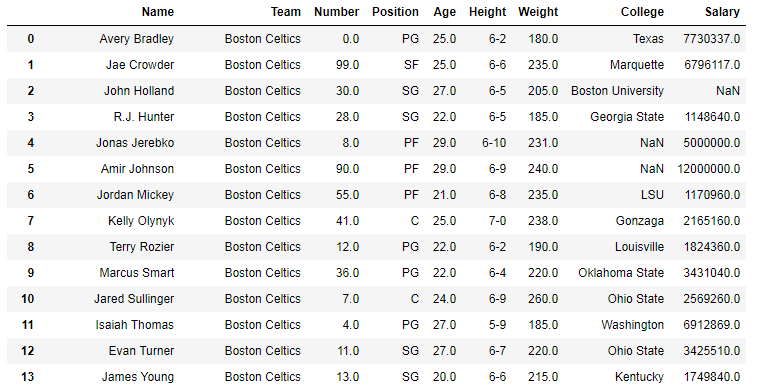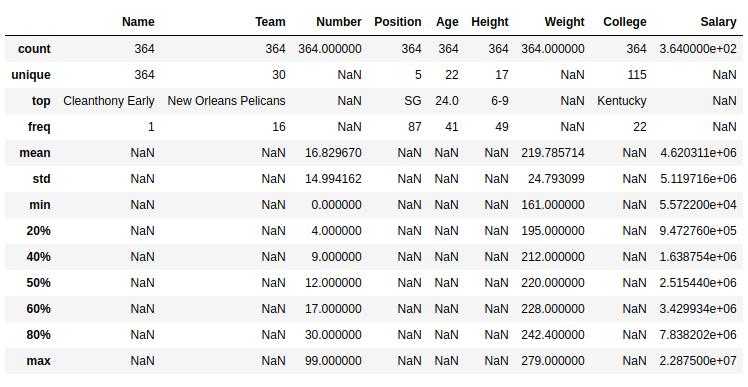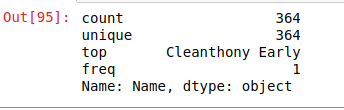# Python | Pandas Dataframe.describe() method

Python is a great language for doing data analysis, primarily because of the fantastic ecosystem of data-centric Python packages. Pandas is one of those packages and makes importing and analyzing data much easier.

Pandas `describe()` is used to view some basic statistical details like percentile, mean, std etc. of a data frame or a series of numeric values. When this method is applied to a series of string, it returns a different output which is shown in the examples below.

Syntax: DataFrame.describe(percentiles=None, include=None, exclude=None)

Parameters:
percentile: list like data type of numbers between 0-1 to return the respective percentile
include: List of data types to be included while describing dataframe. Default is None
exclude: List of data types to be Excluded while describing dataframe. Default is None

Return type: Statistical summary of data frame.

In the following examples, the data frame used contains data of some NBA players. The image of data frame before any operations is attached below.Example #1: Describing data frame with both object and numeric data type

In this example, the data frame is described and [‘object’] is passed to include parameter to see description of object series. [.20, .40, .60, .80] is passed to percentile parameter to view the respective percentile of Numeric series.

 `# importing pandas module  ` `import` `pandas as pd  ` ` `  `# importing regex module ` `import` `re ` `   `  `# making data frame  ` `data ``=` `pd.read_csv(``"https://media.geeksforgeeks.org/wp-content/uploads/nba.csv"``)  ` `   `  `# removing null values to avoid errors  ` `data.dropna(inplace ``=` `True``)  ` ` `  `# percentile list ` `perc ``=``[.``20``, .``40``, .``60``, .``80``] ` ` `  `# list of dtypes to include ` `include ``=``[``'object'``, ``'float'``, ``'int'``] ` ` `  `# calling describe method ` `desc ``=` `data.describe(percentiles ``=` `perc, include ``=` `include) ` ` `  `# display ` `desc `

Output:
As shown in the output image, Statistical description of dataframe was returned with the respective passed percentiles. For the columns with strings, NaN was returned for numeric operations.Example #2: Describing series of strings

In this example, the describe method is called by the Name column to see the behaviour with object data type.

 `# importing pandas module  ` `import` `pandas as pd  ` ` `  `# importing regex module ` `import` `re ` `   `  `# making data frame  ` `data ``=` `pd.read_csv(``"https://media.geeksforgeeks.org/wp-content/uploads/nba.csv"``)  ` `   `  `# removing null values to avoid errors  ` `data.dropna(inplace ``=` `True``)  ` ` `  `# calling describe method ` `desc ``=` `data[``"Name"``].describe() ` ` `  `# display ` `desc `

Output:
As shown in the output image, the behaviour of describe() is different with series of strings.
Different stats were returned like count of values, unique values, top and frequency of occurrence in this case.My Personal Notes arrow_drop_upDeveloper in day, Designer at night GSoC 2019 with Python Software Foundation (EOS Design system)

If you like GeeksforGeeks and would like to contribute, you can also write an article using contribute.geeksforgeeks.org or mail your article to contribute@geeksforgeeks.org. See your article appearing on the GeeksforGeeks main page and help other Geeks.

Please Improve this article if you find anything incorrect by clicking on the "Improve Article" button below.

Article Tags :

1

Please write to us at contribute@geeksforgeeks.org to report any issue with the above content.